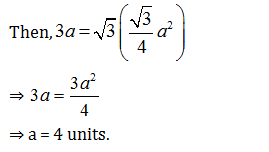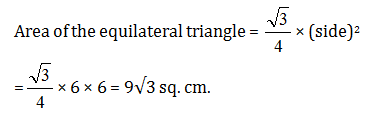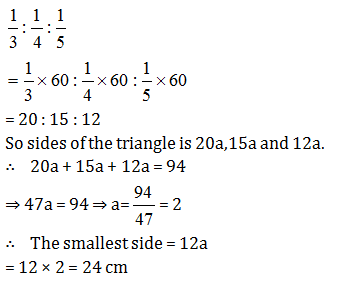Select Page

### CAT 2022 Mensuration Practice Exercise-3

CAT Mensuration questions revolve mostly around 2 important shapes i.e. circles and triangles. Here are some questions based on the concepts of triangle and its types. The key concepts that you need to know for these CAT Mensuration questions are:

• Types of triangles
• Angle and length properties for triangles
• Formulas for finding area and perimeter for different types of triangles

Question 1: If the numerical value of the perimeter of an equilateral triangle is √3 times the area of it. What is the length of each side of the triangle?

(a) 2 unit
(b) 3 unit
(c) 4 unit
(d) 6 unit

The side of the equilateral triangle be a units.Question 2: Each side of an equilateral triangle is 6 cm. Find out its area.

(a) 9√3 sq.cm.
(b) 6√3 sq.cm.
(c) 4√3 sq.cm.
(d) 8√3 sq.cm.Question 3: If a circle with radius 8 cm has same area as triangle with base 8 cm, then find the corre­sponding altitude (in cm) of the triangle.

(a) 12π
(b) 20π
(c) 16π
(d) 32π

Assume the corresponding altitudes of the triangle = a cm

According to the question,

Area of the triangle = Area of the circle

⇒ 1/2 x a × 8 = π × 8 × 8

⇒ a = 2 × 8π = 16π cm.

Question 4: The measures (in cm) of sides of a right angled triangle are given by consecutive integers. What is its area (in cm2)?

(1) 9
(2) 8
(3) 5
(4) 6

Only right angle triangle with consecutive integers is with side 3,4 and 5.

32 + 42 = 52

∴   Base = 3 cm;

Perpendicular = 4 cm

∴   Area of the right angled triangle

= 1/2 × base × height

= 1/2 × 3 × 4 = 6 sq. cm.

Question 5: Sides of a triangle are in the ratio 1/3:1/4:1/5and its perimeter is 94cm. Find the length of the largest side of the triangle?

(a) 18cm
(b) 22.5 cm
(c) 24 cm
(d) 27 cmExtra tips for CAT 2022 Mensuration Questions:

• In order to solve CAT Mensuration questions, revise the basic concepts of triangles and its types- Right angled, equilateral, isosceles, scalene, etc. Each type has different formulas and triangle itself has a set of general formulas for finding different parameters. You can equate these formulas to simplify the solutions in some questions.
• Identify the type of triangle and define the variables appropriately. These type CAT Mensuration questions can also be solved without drawing any diagrams.
• Problems based on sizing the sides as ratios can be solved by simplifying the ratios and then solving using the given parameter.

Get Posts Like This Sent to your Email
Updates for Free Live sessions and offers are sent on mail. Don't worry: we do not send too many emails..:)
Get Posts Like This Sent to your Email
Updates for Free Live sessions and offers are sent on mail. Don't worry: we do not send too many emails..:)